# Performance in Optimization Models: A Comparative Analysis of GAMS, Pyomo, GurobiPy, and JuMP

Posted on: 04 Jul, 2023
Justine Broihan## Introduction

In today’s fast-paced business world, decision-makers face increasingly complex challenges that require sophisticated optimization techniques. With so many modeling languages and frameworks available, it can be difficult to decide which one is the best fit for your specific needs.

We believe that GAMS stands out as a powerful tool for modeling and solving optimization problems. However, we also understand that our clients have many questions about why they should choose GAMS over other popular modeling frameworks such as Pyomo, GurobiPy, or JuMP.

## What this post is about

In this blog post, we’ll provide a comparison of GAMS with these other modeling frameworks, highlighting some of the unique benefits and features that set GAMS apart from the competition. Whether you’re a business leader or a researcher, we’re confident that this post will help you understand why GAMS is a smart choice for modeling and solving complex optimization problems.

One key aspect of any modeling language is how it handles sparse multidimensional data structures. In this blog post, we’ll explore how each of the modeling frameworks - Pyomo, GurobiPy, JuMP, and GAMS - approach this issue and how each approach performs.

It’s worth noting that each of these modeling languages is a capable and successful tool for modeling and solving optimization problems. However, by looking closely at their individual strengths and weaknesses, we hope to provide our users with a better understanding of how GAMS stands out as a powerful and versatile modeling language.

## Problem Characteristics we face in Operations Research

Many optimization problems are subject to a particular structure in which the data cube has a lot of zeros and only a few non-zeros, a characteristic referred to as sparse. In optimization problems, it is often necessary to account for complex mappings of indices to subsets. For example, in the complex world of supply chain management, the sets of products, production units, production plants, distribution centers, and customers are the building blocks of a model. The uniqueness of each product often dictates that only a subset of production units possess the capability to manufacture it. Moreover, at any given production plant, only a subset of all available production units may be accessible. Adding to the complexity, the location of a production plant further narrows down the options for distribution centers, as only a specific subset would be considered suitable for storing products. And finally, only certain distribution centers are considered to deliver products to the customers.

Such a typical structure is best represented by maps. We continue to work with an abstraction (sets $\mathcal{I}$, $\mathcal{J}$, $\mathcal{K}$, $\mathcal{L}$, and $\mathcal{M}$) rather than a real world example to focus on the core of the sparsity issue. For example, a map $\mathcal{IJK}$ is a subset of the Cartesian product $\mathcal{I} \times \mathcal{J} \times \mathcal{K}$. Sparsity of $\mathcal{IJK}$ means that $|\mathcal{IJK}|\ll|\mathcal{I} \times \mathcal{J} \times \mathcal{K}|$. Unlike dictionaries where key and value are clearly specified, maps can be used with different key (combinations). So in one situation, the map provides the set elements $j$ and $k$ for a given $i$, in other situations the same map provides set elements $i$ for a given $j$ and $k$. We’ll explore how each of the modeling languages we’ve mentioned handles this sparse structure and complex mappings of indices, and how they perform with growing problem size with the following abstract and partial but typically structured model algebra:

$$\min F = 1$$

$$\sum_{(j,k):(i,j,k) \in \mathcal{IJK}} \ \sum_{l:(j,k,l) \in \mathcal{JKL}} \ \sum_{m:(k,l,m) \in \mathcal{KLM}} x_{i,j,k,l,m} \ge 0 \hspace{1cm} \forall \ i \in \mathcal{I}$$

$$x_{i,j,k,l,m} \ge 0 \hspace{1cm} \forall \ (i,j,k) \in \mathcal{IJK}, l:(j,k,l) \in \mathcal{JKL}, m:(k,l,m) \in \mathcal{KLM}$$

## General-Purpose Programming Language vs Domain Specific Modeling Languages

One of the key differences between GAMS and the other modeling frameworks we’ve mentioned is that GAMS is a domain-specific language, whereas the rest are based on general-purpose programming languages. This means that GAMS is specifically designed to represent algebraic models in a way that computers can execute the model and users are still able to easily read the model. In contrast, modeling frameworks like Pyomo, JuMP, and GurobiPy rely on general programming conventions to represent algebraic models as effectively as possible. Thanks to its domain-specific language, GAMS allows us to write the model using only a few lines of code that are very similar to the algebraic formulation.

If we use Pyomo to represent the same model, we end up with considerably more lines of code and nested operations. This difference can have a significant impact on the readability of the model and thus on the time and effort required to develop, test, and maintain complex optimization models. An excellent illustration of the simplicity and user-friendliness of GAMS as a modeling language can be found by comparing the variable declaration ($x_{i,j,k,l,m}$) syntax in GAMS with Pyomo’s syntax.

    model.x = pyo.Var(
[
(i, j, k, l, m)
for (i, j, k) in model.IJK
for (jj, kk, l) in model.JKL
if (jj == j) and (kk == k)
for (kkk, ll, m) in model.KLM
if (kkk == k) and (ll == l)
],
domain=pyo.NonNegativeReals,
)


vs

Positive variable x(i,j,k,l,m);


The reason for this significant difference in variable declaration between Pyomo and GAMS is that GAMS automatically takes care of generating variables only for the relevant combinations of indices based on the algebraic formulation, while in Pyomo, we need to carefully define the relevant combinations of variable indices ourselves. This feature is particularly useful when working with a large multidimensional index space, where generating all possible combinations of indices would be computationally expensive and unnecessary. GAMS quietly handles this task in the background, allowing us to focus on the formulation of the model.

## Isn’t more flexibility always better?

Figure 1 presents the time required to generate a model instance for the exemplary mathematical model with Pyomo, as a function of problem size, specifically the growing size of set $\mathcal{I}$. The figure demonstrates the model generation time for an implementation of the model where all variables are defined as the Cartesian product of all indices without carefully defining only the relevant variables. In contrast, Figure 1 also presents the model generation time for an implementation where only relevant variables are carefully defined. We report the minimum model generation time across multiple runs for every problem size data point. Comparing the model generation time for the two implementations emphasizes the importance of precise variable definition in mathematical optimization. The model generation time for the Cartesian product implementation increases drastically with problem size, while the carefully defined variable implementation maintains a moderate model generation time.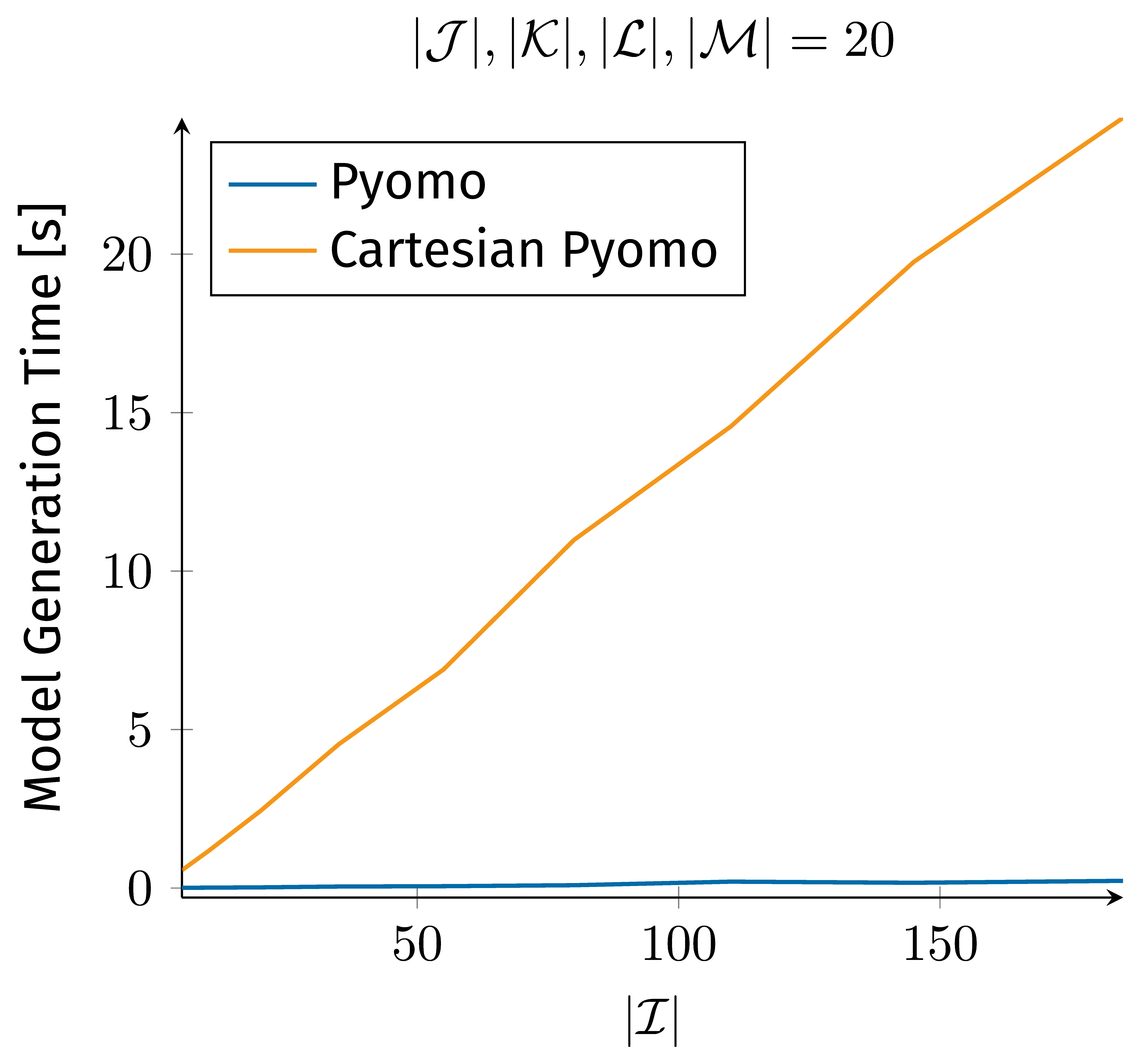Figure 1. Model generation time for Cartesian and carefully defined variable definitions.

## But General-Purpose Programming Languages, like Python, are so much more flexible than Domain Specific Languages like GAMS

While it’s true that general-purpose programming languages offer more flexibility and control, it’s important to consider the trade-offs. With general-purpose languages like Python and Julia, a straightforward implementation closely aligned with the mathematical formulation is often self-evident and easier to implement, read, and maintain, but suffers from inadequate performance. In attempting to balancing the simplicity of code representing a mathematical model and its performance, trade-offs become necessary.

As we’ve seen in the example of GAMS versus Pyomo, having more control sometimes means taking on more responsibility, such as manually generating only relevant variables. Flexibility is also a double-edged sword. While it offers many different ways to accomplish a task, there is also the risk of implementing a solution that is not efficient. And determining the optimal approach is a challenging task in itself. All of the discussed modeling frameworks allow a more or less and depending on personal taste intuitive implementation of our example’s model. However, intuitive solutions do not always turn out to be efficient. With additional research and effort, it is possible to find alternative implementations that outperform the intuitive approach, as Figure 2 presents for JuMP.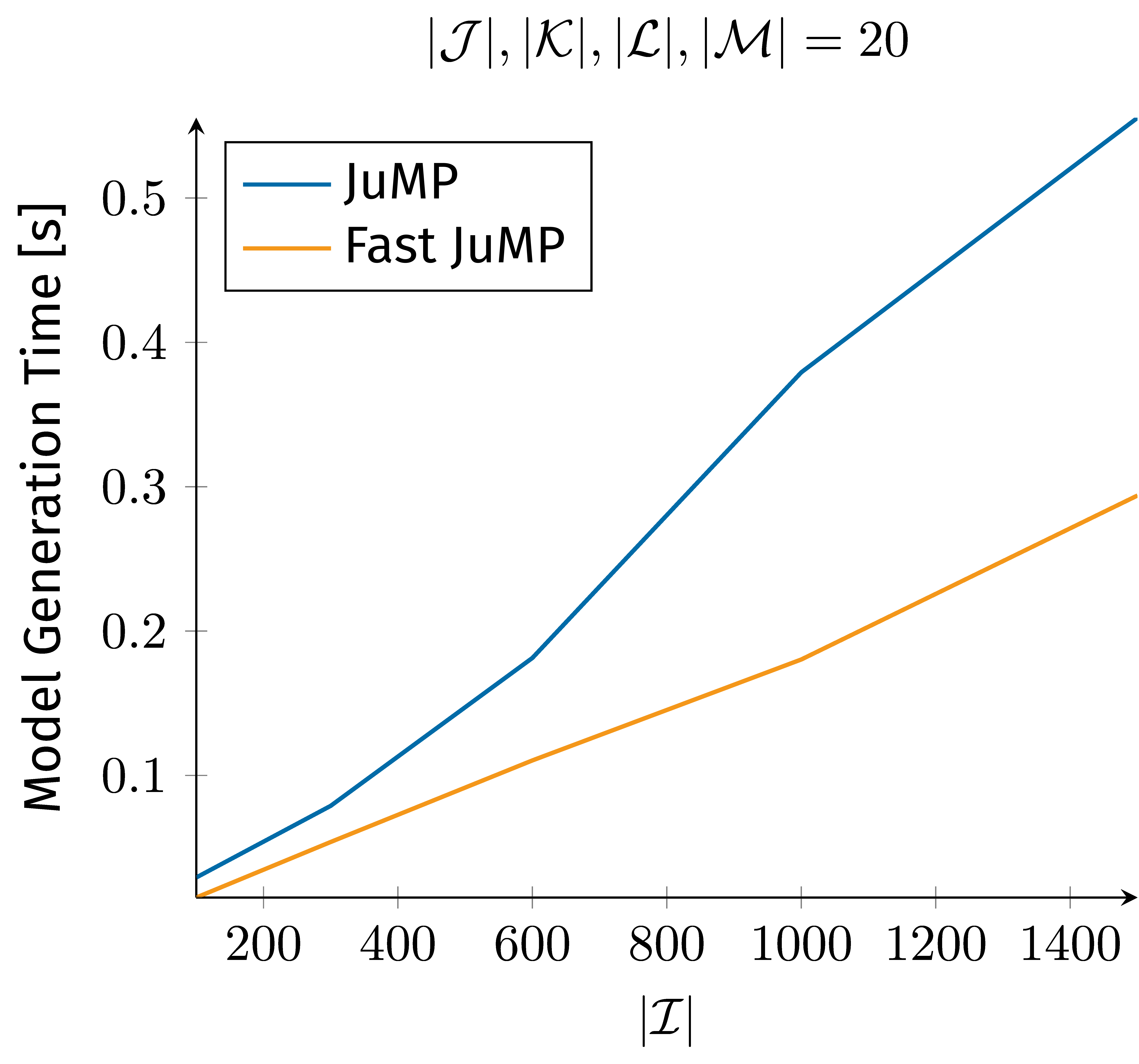Figure 2. Compared model generation times for an low and high performing implementation in JuMP.

We see the same principle that applies to JuMP when it comes to modeling frameworks like GurobiPy and Pyomo with the underlying language Python. Figure 3 outlines the difference in intuitive versus optimized data structures for model representation with Pyomo and GurobiPy.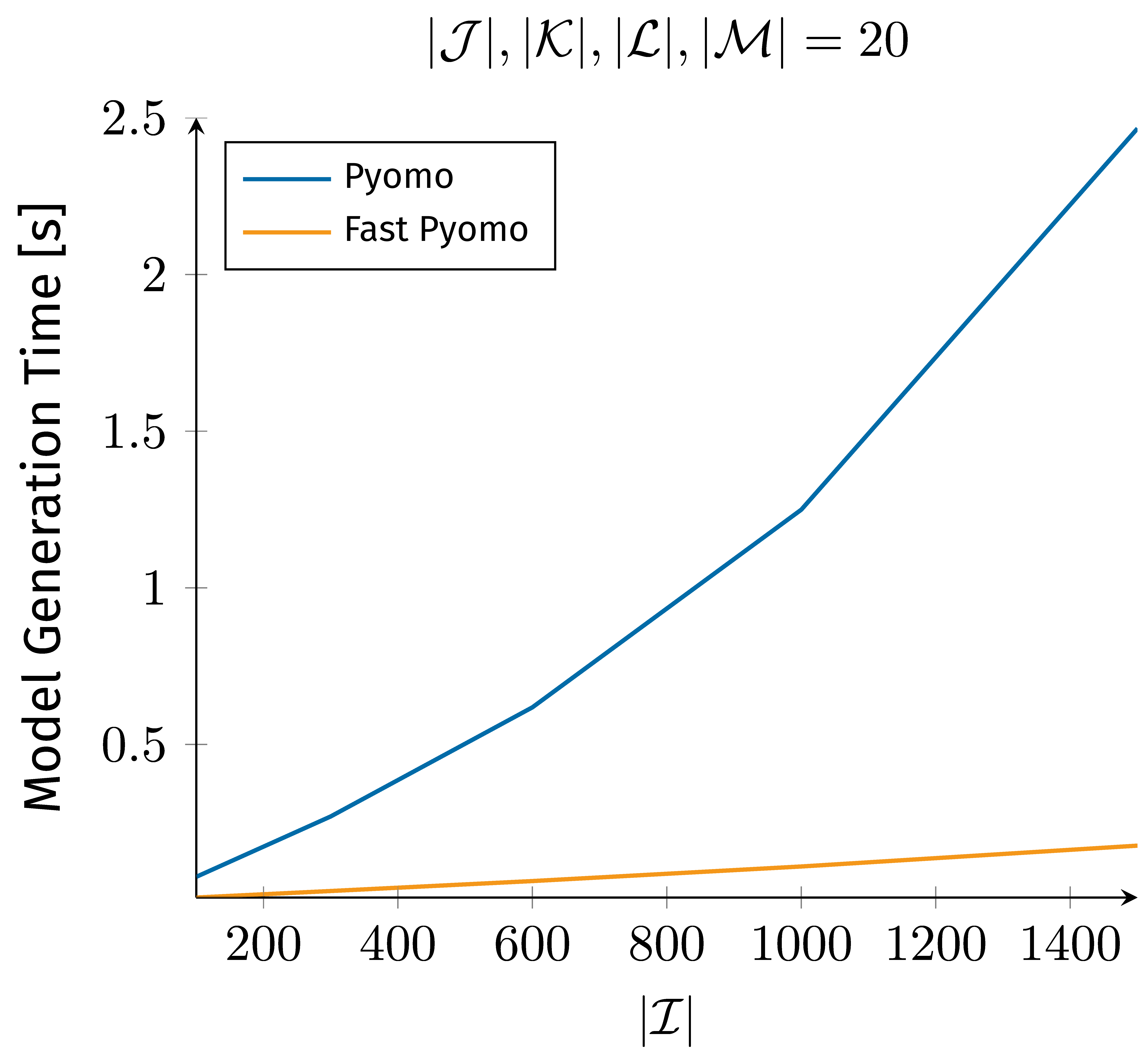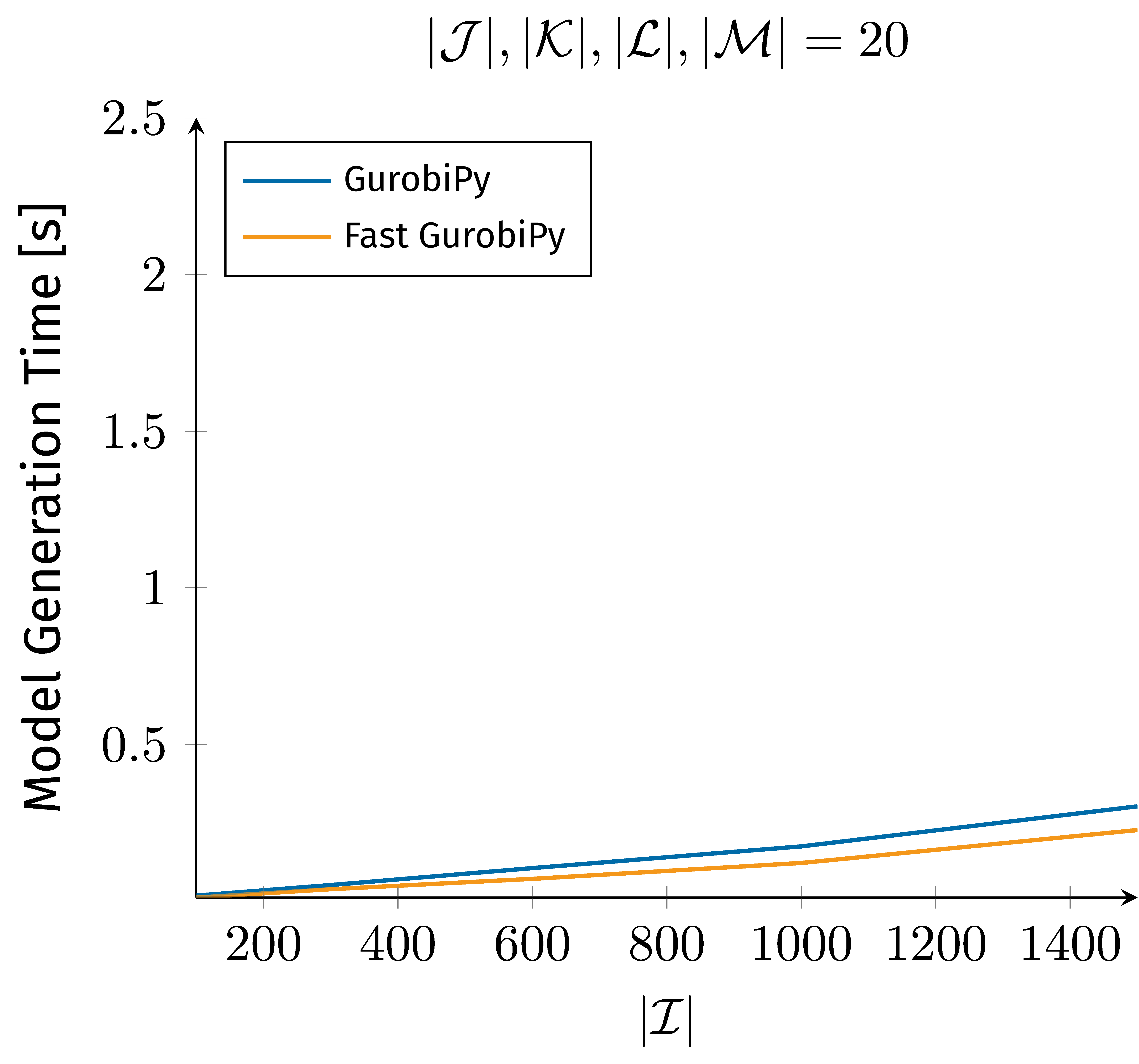As we’ve seen in Figures 2 and 3 with our Pyomo, JuMP, and GurobiPy examples, relying solely on intuition and a straightforward implementation may not always lead to the most efficient solution. It takes a significant amount of research and effort to find ways to optimize the model representation and improve its performance with respect to model generation time. While model and parameter tuning is always part of optimization model development it should not obscure the mathematical foundation and should not be in the way of maintenance and further development. Especially during the early development phase, it is crucial to have the ability to easily evaluate changes to the model and engage in rapid prototyping without the cumbersome burden of tuning with each modification. This is where domain-specific languages like GAMS shine. GAMS provides with its specialized syntax the bases for built-in code optimization that in modeling frameworks built on top of a general-purpose programming language needs to be manually performed by the user.

Now that we have discussed how those languages are used, let’s compare their performances. In order to evaluate the performance of GAMS, Pyomo, GurobiPy, and JuMP, we conduct a study using generated dataset for sets $\mathcal{I}$, $\mathcal{J}$, $\mathcal{K}$, $\mathcal{L}$, and $\mathcal{M}$ with a cardinality of $N$ for set $\mathcal{I}$ and cardinality of $O$ for sets $\mathcal{J}$, $\mathcal{K}$, $\mathcal{L}$, and $\mathcal{M}$. Random multidimensional sets $\mathcal{IJK}$, $\mathcal{JKL}$, and $\mathcal{KLM}$ are generated with a 5% chance of $(i,j,k)$, $(j,k,l)$, and $(k,l,m)$ being in $\mathcal{IJK}$, $\mathcal{JKL}$, $\mathcal{KLM}$, respectively, for the full Cartesian product of $\mathcal{I}\times\mathcal{J}\times\mathcal{K}$, $\mathcal{J}\times\mathcal{K}\times\mathcal{L}$, and $\mathcal{K}\times\mathcal{L}\times\mathcal{M}$. As we increase $N$, the cardinality of $\mathcal{I}$ and $\mathcal{IJK}$ also increases. We measure the time that each language needs to generate and solve with a solver time limit of zero seconds the presented model, with each language working with the same data set. We report the minimum model generation time achieved by each language across a statistically relevant number of runs per data set.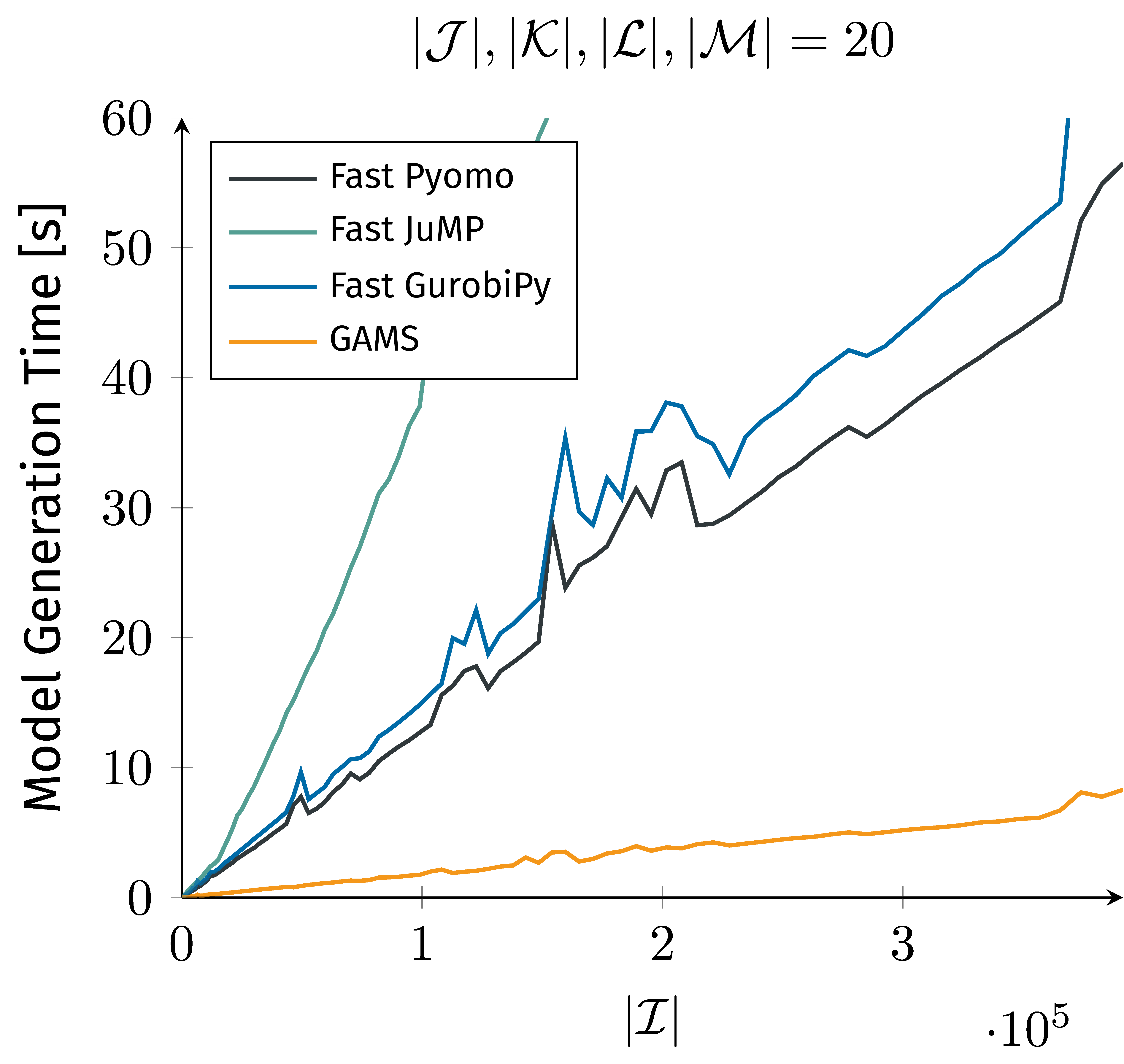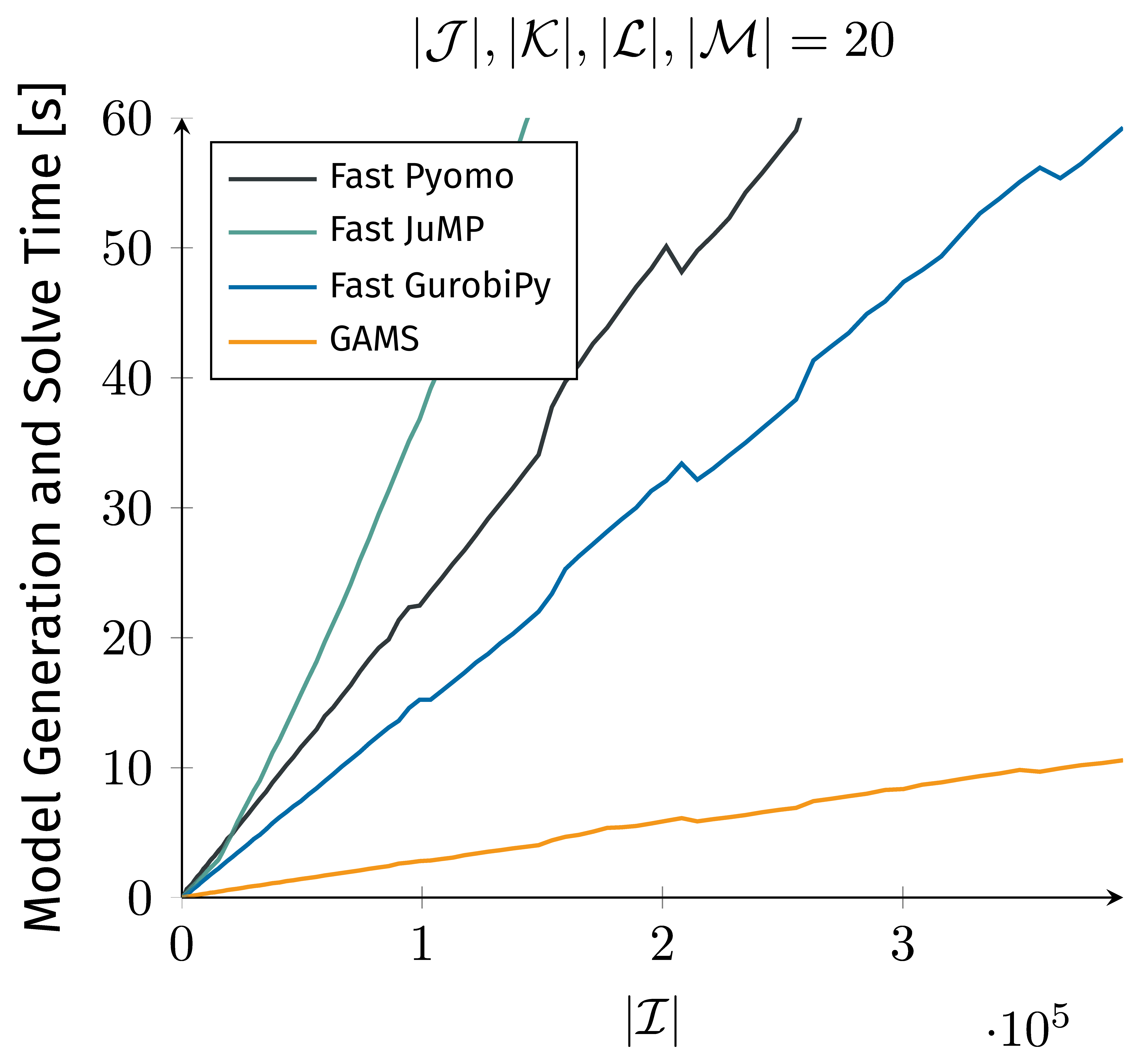According to the obtained results in Figure 4, there is a huge difference in performance for the introduced languages. While we observe linear growth for GurobiPy, JuMP, and Pyomo, the model generation time for GAMS does not increase as significantly.

## But why does GAMS outperform Pyomo, JuMP, and GurobiPy?

The reason for GAMS superior performance in this example is the use of relational algebra. While other optimization software such as Pyomo, GurobiPy, and JuMP require iterating over all elements of a set, GAMS uses relational algebra to process complex queries efficiently. Relational algebra originates from database theory and allows complex database queries to be processed very efficiently without the need to iterate over all database entries. As a result, GAMS can handle large-scale optimization problems more efficiently and effectively than many other modeling languages.

## But why do I care about model generation?

When it comes to mathematical optimization, the significance of model generation time should not be underestimated. While it may be tempting to solely focus on the performance of the solver, the truth is that the time taken for model generation can greatly impact the overall efficiency and practicality of the optimization process. Admittedly, if the solver consumes the majority of the time, the relevance of model generation diminishes to some extent. However, feedback from our customers consistently emphasize the importance of considering performance beyond solving alone. A notable example that highlights this perspective is conveyed by Abhijit Bora, who shared PROS Inc. experience in transferring their optimization model to GAMS.

Our decision to reimplement our large optimization model using GAMS has yielded exceptional results, surpassing all previous modeling technologies. With an extraordinary improvement of 300%, this accomplishment holds immense significance for us. It unlocks the door to unprecedented possibilities in optimization. We can now embrace a 360-day horizon, a long-desired feature that was once deemed unattainable but has become a reality.

– Abhijit Bora, Senior Principal Software Engineer at PROS Inc.

Other recent research also focuses on efficiency of model generation. The paper Linopy: Linear optimization with n-dimensional labeled variables1 compares some open source modeling frameworks but unfortunately chose a dense model (the knapsack problem) as a benchmark problem. In our experience dense models are extremely rare in practice and don’t really represent a challenge with respect to model generation. The ongoing study Computational Performance of Algebraic Modeling Languages Under Practical Use Cases at Carnegie Mellon University2 also analyzes different modeling frameworks with an emphasis on model generation performance.

## Let’s summarize

GAMS has proven to be a powerful optimization tool due to its use of relational algebra and efficient handling of complex variable definitions. Its mathematical notation also allows for intuitive and readable model implementations. While the choice between a domain-specific language like GAMS and a general-purpose programming language package like Pyomo ultimately depends on the problem at hand, it’s important to consider the trade-offs between control, flexibility, and efficiency. With careful consideration and effort, either type of language can achieve optimal results.

For anyone interested you can find the full code used for this analysis in our GitHub repository.

This post has been updated on July 13, 2023.

A previous version of this post included content (quotes from public forums3 4 and an acknowledgement) that may have conveyed the false impression that JuMP and Pyomo developers collaborated in creating this blog post or approved of its content. We want to clarify that this was not the case and have since removed this content.

1. Hofmann, F., (2023). Linopy: Linear optimization with n-dimensional labeled variables. Journal of Open Source Software, 8(84), 4823, https://doi.org/10.21105/joss.04823  ↩︎

2. Kompalli, S., Merakli, M., Ammari, B. L., Qian, Y., Pulsipher, J. L., Bynum, M., Furman, K. C., Laird, C. D. (2023). Computational Performance of Algebraic Modeling Languages Under Practical Use Cases. Carnegie Mellon University, Annual Review Meeting, March 6-7, 2023. ↩︎